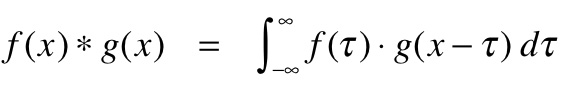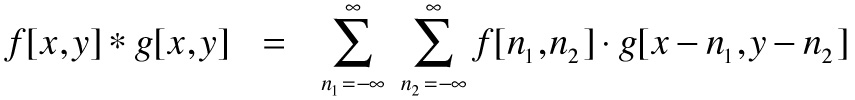# Spatial convolution

Applet: Katie Dektar
Text: Marc Levoy
Technical assistance: Andrew Adams

Convolution is an operation on two functions f and g, which produces a third function that can be interpreted as a modified ("filtered") version of f. In this interpretation we call g the filter. If f is defined on a spatial variable like x rather than a time variable like t, we call the operation spatial convolution. Convolution lies at the heart of any physical device or computational procedure that performs smoothing or sharpening. Applied to two dimensional functions like images, it's also useful for edge finding, feature detection, motion detection, image matching, and countless other tasks. Formally, for functions f(x) and g(x) of a continuous variable x, convolution is defined as:where * means convolution and · means ordinary multiplication. For functions of a discrete variable x, i.e. arrays of numbers, the definition is:Finally, for functions of two variables x and y (for example images), these definitions become:andIn digital photography, the image produced by the lens is a continuous function f(x,y), Placing an antialiasing filter in front of the sensor convolves this image by a smoothing filter g(x,y). This is the third equation above. Once the image has been recorded by a sensor and stored in a file, loading the file into Photoshop and sharpening it using a filter g[x,y] is the fourth equation.

Despite its simple definition, convolution is a difficult concept to gain an intuition for, and the effect obtained by applying a particular filter to a particular function is not always obvious. In this applet, we explore convolution of continuous 1D functions (first equation) and discrete 2D functions (fourth equation).

#### Convolution of 1D functions

On the left side of the applet is a 1D function ("signal"). This is f. You can draw on the function to change it, but leave it alone for now. Beneath this is a menu of 1D filters. This is g. If you select "custom" you can also draw on the filter function, but leave that game for later. At the bottom is the result of convolving f by g. Click on a few of the filters. Notice that "big rect" blurs f more than "rect", but it leaves kinks here and there. Notice also that "gaussian" blurs less than "big rect" but doesn't leave kinks.

Both functions (f and g) are drawn as if they were functions of a continuous variable x, so it would appear that this visualization is showing convolution of continuous functions (first equation above). In practice the two functions are sampled finely and represented using 1D arrays. These numbers are connected using lines when they are drawn, giving the appearance of continuous functions. The convolution actually being performed in the applet's script is of two discrete functions (second equation above).

Whether we treat the convolution as continuous or discrete, its interpretation is the same: for each position x in the output function, we shift the filter function g left or right until it is centered at that position, we flip it left-to-right, we multiply every point on f by the corresponding point on our shifted g, and we add (or integrate) these products together. The left-to-right flipping is because, for obscure reasons, the equation for convolution is defined as g[x-k], not g[x+k] (using the 2nd equation as an example).

#### An alternative way to think about convolution

If this procedure is a bit hard to wrap your head around, here's an equivalent way to describe it that may be easier to visualize: at each position x in the output function, we place a copy of the filter g, centered left-to-right around that position, flipped left-to-right, and scaled up or down according to the value of the signal f at that position. After laying down these copies, if we add them all together at each x, we get the right answer!

To see this alternative way of understanding convolution in action, click on "animate", then "big rect". The animation starts with the original signal f, then places copies of the filter g at positions along f, stretching them vertically according to the height of f at that position, then adds these copies together to make the thick output curve. Although the animation only shows a couple dozen copies of the filter, in reality there would need to be one copy for every position x. In addition, for this procedure to work the sum of copies must be divided by the area under the filter function, a processing called normalization. Otherwise, the output would be higher or lower than the input, rather than simply being smoothed or sharpened. For all the filters except "custom", normalization is performed for you just before drawing the thick output curve. For the "custom" filter, see below.

Once you understand how this works, try the "sharpen" or "shift" filters. The sharpen filter replaces each value of f with a weighted sum of its immediate neighbors, but subtracting off the values of neighbors a bit further away. The effect of these subtractions is to exaggerate features in the original signal. The "Sharpen" filter in Photoshop does this; so do certain layers of neurons in your retina. The shift filter replaces each value of f with a value of f taken from a neighbor some distance to the right. (Yes, to the right, even though the spike in the filter is on the left side. Remember that convolution flips the filter function left-to-right before applying it.)

Finally, click on "custom" and try drawing your own filter. If the area under your filter is more or less than 1.0, the output function will jump up or down, respectively. To avoid this, click on "normalize". This will scale your filter up or down until its area is exactly 1.0. By the way, if you animate the application of your custom filter, the scaled copies will only touch the corresponding point on the original function if your custom filter reached y=1.0 at its x=0 position. Regardless, if your filter is normalized, the output function will be of the right height.

#### Convolution of 2D functions

On the right side of the applet we extend these ideas to two-dimensional discrete functions, in particular ordinary photographic images. The original 2D signal is at top, the 2D filter is in the middle, depicted as an array of numbers, and the output is at the bottom. Click on the different filter functions and observe the result. The only difference between "sharpen" and "edges" is a change of the middle filter value from 9.00 to 8.00. However, this change is crucial, as you can see. In particular, the sum of all non-zero filter values in "edges" is zero. Therefore, for positions in the original signal that are smooth (like the background), the output of the convolution is zero (i.e. black). The filter "hand shake" approximates what happens in a long-exposure photograph, in this case if the camera's aim wanders from upper-left to lower-right during the exposure.

Finally, click on "identity", which sets the middle filter tap (as positions in a filter are sometimes called) to 1.0 and the rest to 0.0. It should come as no surprise that this merely makes a copy of the original signal. Now click on "custom", then click on individual taps and enter new values for them. As you enter each value, the convolution is recomputed. Try creating a smoothing filter, or a sharpening filtering. Or starting with "identity", change the middle tap to 0.5, or 2.0. Does the image scale down or up in intensity? The applet is clipping outputs at 0.0 (black) and 255.0 (white), so if you try to scale intensites up, the image will simply saturate - like a camera if your exposure were too long. Try putting 1.0's in the upper-left corner and the lower right corner, setting everything else to 0.0. Do you get a double image? As with the custom 1D filter, if your filter values don't sum to 1.0, you might need to press "normalize". Unless you're doing edge finding, in which case they should sum to 0.0.